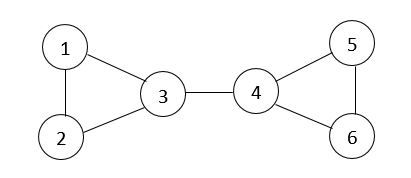# World War in Jupiter

Limits 1s, 512 MB

The peaceful days for the people of Jupiter is over. It's world war 3! Mr Drump, the president of Twinland wants to destroy all the countries and take over the whole galaxy. He has made some dangerous bombs which contains TBA virus. TBA virus is very dangerous, if it affects any of the country then the country get destroyed. But he has already used most of his bombs and now he's only left with one. He wants to drop this last one optimally.

The spreading process of TBA virus is quite different. Initially, TBA virus stays where the bomb has been dropped. Then it follows an unusual algorithm for spreading.

1. Let's assume $cur$ is the country where the virus is currently in.

2. It destroys the country $cur$ and it takes $K$ minutes to do so.

3. Then it moves randomly to one of the neighboring countries of $cur$ which is not destroyed yet and it takes 0 minutes. Then it repeats the process from step 1) again.

4. If there is no neighboring country of $cur$ which is not destroyed yet then the virus destroys itself.

Mr Drump has sent a spy to explore the Jupiter. Based on his report Mr Drump will initiate the attack. But he wants to verify if the spy truly visited the Jupiter or not. So he asked him a question.

How many triplets of $(P,U,V)$ are there such that after dropping the bomb on country $P$, no matter what path the virus follows, if both the country $U$ and $V$ get destroyed then $T(U)$ will always be smaller than $T(V)$. Here $T(i)$ is the time when country i get destroyed.

Now Mr Drump needs your help to verify the answer. Can you help him?

## Input

The first line contains three integers $N$, $M$ and $K$. $N$ is the number of country in Jupiter. $M$ is the number of neighboring relations and $K$ is already explained above.

Then $M$ line follows. Each of which contains two integers $a$ and $b$ meaning that the country $a$ and $b$ are neighbors with each other.

Constraints:

• $1 ≤ N,M ≤ 10^5$

• $1 ≤ K ≤ 10^9$

• $1 ≤ a,b ≤ N$

## Output

Print a single integer, the answer to the question of Mr Drump. As this value might become huge, you need to print it with modulo $1000000007$.

## Sample

InputOutput
6 7 5
1 2
2 3
1 3
3 4
4 5
6 5
4 6
24


In the first test case one of the possible triplet is $(1,3,6)$.After placing the bomb on city 1, the virus will first destroy the city 1 at time 0. Then it moves to city 2 (randomly chosen from 2 & 3) and destroy it at time 5. Then moves to city 3 and destroy it at time 10. Again moves to city 4 and destroy it at time 15. Now moves to city 5 (randomly chosen from 5 & 6) and destroy it at time 20. Finally it moves to city 6 and destroy it at time 25. As there is no other neighbors of city 6 which is not destroyed yet, so the virus destroy itself. And the process ends here. So here the virus followed the path (1 -> 2 -> 3 -> 4 -> 5 -> 6). But the virus could also follow the path (1 -> 2 -> 3 -> 4 -> 6 -> 5) or (1 -> 3 -> 4 -> 5-> 6) or (1 -> 3 -> 4 -> 6 -> 5). In each of this path T(3) is always smaller than T(6). There is not any single path where $T(6) >= T(3)$. So $(1,3,6)$ is a valid triplet.

P, U, V must be unique to be considered as a valid triplet.